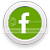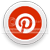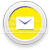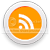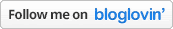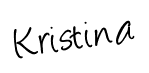I shared our Christmas Tree addition activity with you a couple weeks ago, and to practice math facts once more we brought out some cars to reinforce Number Bonds.
Using duct tape, I made a triangle on the floor and then drew out triangles with the numbers to create and solve the equation.  In the upside down triangle, one angle is blank and the other angle contains a number to help solve the equation.  The angle pointing down contains the sum.

## Number Bonds Math Activity

All the cars begin at the bottom of the upside down triangle.

In the picture below, we started off with 9 cars (the sum).  T drove 6 cars to one corner to figure out the problem: ___ + 6 = 9, thus 3 cars were left and so it was our answer.By moving x number of cars to one side of the triangle, he was able to see that the missing number was the number of cars left at the bottom of the triangle.

I'd love for you to follow along so you never miss out on one of our fun activities we share at School Time Snippets!